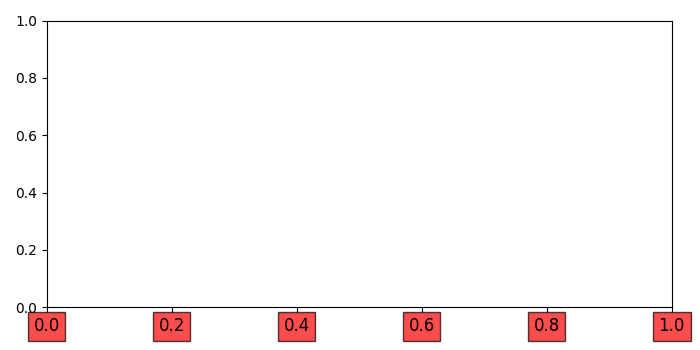# How to put xtick labels in a box matplotlib?

To put xtick labels in a box, we can take the following steps

## Steps

• Create a new figure or activate an existing figure.

• Get the current axis of the figure.

• Set the left and bottom position of the axes.

• Set the position of the spines, i.e., bottom and left.

• To put xtick labels in a box, iterate the ticklabels and use set_bbox() method.

• To display the figure, use Show() method.

## Example

import matplotlib.pyplot as plt

plt.rcParams["figure.figsize"] = [7.00, 3.50]
plt.rcParams["figure.autolayout"] = True

plt.figure()

ax = plt.gca()

ax.xaxis.set_ticks_position('bottom')
ax.yaxis.set_ticks_position('left')

ax.spines['bottom'].set_position(('data', 0))
ax.spines['left'].set_position(('data', 0))

for label in ax.get_xticklabels():
label.set_fontsize(12)
label.set_bbox(dict(facecolor='red', edgecolor='black', alpha=0.7))

plt.show()

## Output

It will produce the following output −